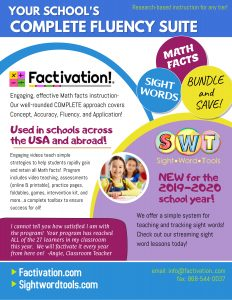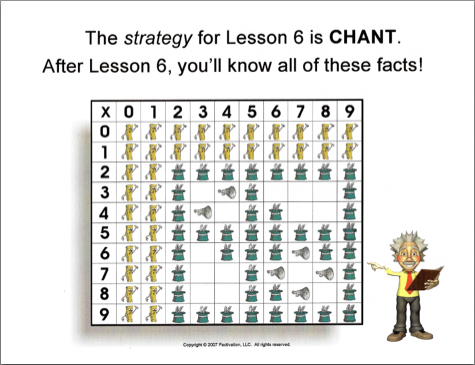##### Print or Share Flyer##### Explore Lessons![spacer]

# Introduce the Lesson 6 Focus Facts

## Fact Tracker for This LessonUse the Fact Tracker (Multiplication chart) below for goal setting and increased motivation! Facts from the current and previous lessons are marked with a strategy icon (rule, trick, chant, or connection). Students are excited to see how many facts they will know after completing this lesson!
[spacer][spacer][spacer] [hr]

## Visual Aids for This Lesson

Project and discuss the visual aids that follow. They represent each of the focus facts from this lesson. The first set illustrates the fact as groups of objects. The second set illustrates the facts as colorful arrays.

### Multiplication Arrays

[spacer][spacer][spacer][spacer][spacer][spacer][hr]

## Printable Concept Builders for This Lesson

Using the Concept Builders that follow, students closely examine individual focus facts from this lesson. For each fact presented, students will:

• Construct Models
• Illustrate the Commutative Property
• Interpret Arrays
• Build Fact Families (Connect to Division)[spacer]

### CCSS 3.OA.A.1, 3.OA.A.2, 3.OA.B.5

[spacer][spacer][spacer][spacer][spacer][spacer][hr]

## Concept Mats and Arrays

### Model Multiplication

Before beginning the lesson, spend some time building each of the Lesson 6 focus facts. The Concept Mats and Arrays below can be printed and reused with each lesson. Using simple manipulatives (goldfish crackers, M&M’s, and pennies), students can create both models and arrays to illustrate each fact. This activity can be done whole-group or as a Multiplication center.

### Connect to Division

Use the Concept Mats to build the Division counterparts! Give students the total number first and tell them how many groups to divide them into. Ask, “How many objects are in each group?”[spacer]